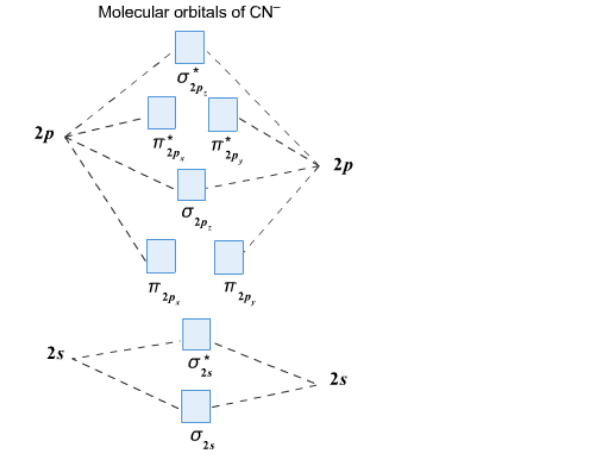Chemistry Practice Problems Heteronuclear Diatomic Molecules Practice Problems Solution: Complete this molecular orbital diagram for CN– th...

🤓 Based on our data, we think this question is relevant for Professor Geissler's class at CAL.

# Solution: Complete this molecular orbital diagram for CN– then determine the bond order. Note that the 1s orbital is not shown in this problem.

###### Problem

Complete this molecular orbital diagram for CN then determine the bond order. Note that the 1s orbital is not shown in this problem.Heteronuclear Diatomic Molecules

Heteronuclear Diatomic Molecules

#### Q. Utilize the molecular orbital diagram below to answer the following questions.   How many electrons are present in antibonding molecular orbitals?  ...

Solved • Wed Mar 09 2016 13:21:41 GMT-0500 (EST)

Heteronuclear Diatomic Molecules

#### Q. Use the molecular orbital diagram to figure out the electronic configuration for CN. Which of the following statements is correct?a) CN is diamagnetic...

Solved • Wed Mar 02 2016 11:48:43 GMT-0500 (EST)

Heteronuclear Diatomic Molecules

#### Q. Use the molecular orbital energy diagram below for the next three questions. How many unpaired electrons are in O 2+? a. 0       b. 1       c. 2    ...

Solved • Fri Feb 19 2016 12:18:35 GMT-0500 (EST)

Heteronuclear Diatomic Molecules

#### Q. Which of the following mixtures of atomic orbitals best describes the σ bonding orbitals in HeH+?   a. s + s b. s – s c. pz + pz d. px – pz e. p...

Solved • Fri Feb 19 2016 12:14:51 GMT-0500 (EST)